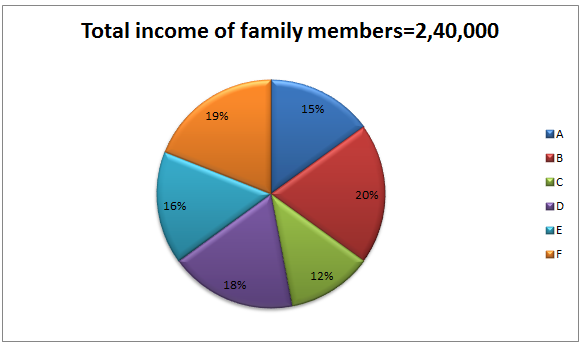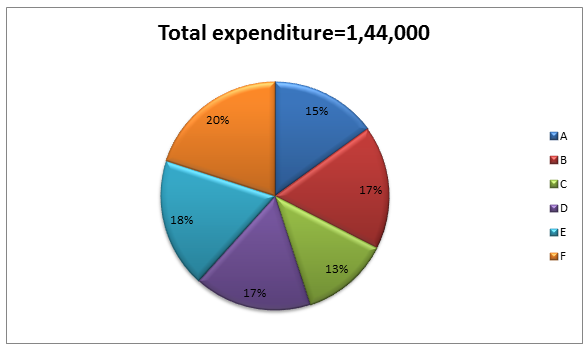## Questions On Quantitative Techniques SMQTQ011Direction: Study the following bar chart carefully and answer the questions given beside.Q.1 What is the difference between the Income of F and the expenditure of A?

A. 36000
B. 21000
C. 30000
D. 24000
E. 27000

Q.2 What is the ratio of total income of A and B together and the total expenditure of E and F together?

A. 149:151
B. 123:150
C. 175:114
D. 100:93
E. 114:175

Q.3 Find the total saving of B and D.

A. 45200
B. 42640
C. 43950
D. 46250
E. None of these

Q.4 Find the average income of A, C and D.

A. 36000
B. 45000
C. 28800
D. 35000
E. 30000

Q.5 If the income of F is increased by 10% and the expenditure increased by 5%. Find the saving.

A. 14325
B. 16250
C. 19920
D. 15650
E. 18520

Solution:

1. Ans. D.

Solution:

=240000×19% - 144000×15%
=45600-21600
=24000

2. Ans. C.

Solution:

Income of A and B: Expenditure of E and F
=240000×(15+20)%:144000×(20+18)%
=240000×35%:144000×38%
=240×35:144×38
=8400:5472
=175:114

3. Ans. E.

Solution:

Saving = Income-Expenditure
=240000×(20+18)% - 144000×(17+17)%
=240000×38% - 144000×34%
=91200-48960
=42240

4. Ans. A.

Solution:

=240000×(15+12+18)%/3
=240000×45%/3
=36000

5. Ans. C.

Solution:

Now the income of F= 240000×19%×110/100
=50160
The expenditure of F= 144000×20%×105%
=30240
Saving= 50160-30240
=19920